Next: Orbital Angular Momentum Up: White-Dwarf Stars Previous: White-Dwarf Stars

### Exercises

1. Consider a particle of massmoving in a three-dimensional isotropic harmonic oscillator potential of force constant. Solve the problem via the separation of variables, and obtain an expression for the allowed values of the total energy of the system (in a stationary state).
2. Repeat the calculation of the Fermi energy of a gas of fermions by assuming that the fermions are massless, so that the energy-momentum relation is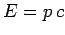.
3. Calculate the density of states of an electron gas in a cubic box of volume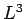, bearing in mind that there are two electrons per energy state. In other words, calculate the number of electron states in the intervalto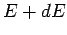. This number can be written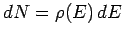, where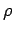is the density of states.
4. Repeat the above calculation for a two-dimensional electron gas in a square box of area.
5. Given that the number density of free electrons in copper is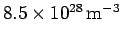, calculate the Fermi energy in electron volts, and the velocity of an electron whose kinetic energy is equal to the Fermi energy.
6. Obtain an expression for the Fermi energy (in eV) of an electron in a white dwarf star as a function of the stellar mass (in solar masses). At what mass does the Fermi energy equal the rest mass energy?Next: Orbital Angular Momentum Up: White-Dwarf Stars Previous: White-Dwarf Stars
Richard Fitzpatrick 2010-07-20